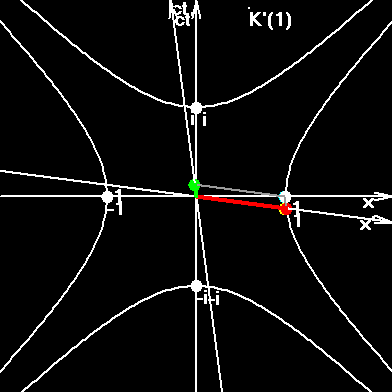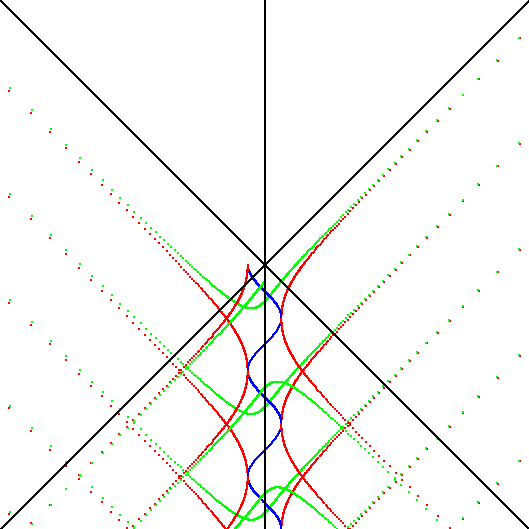# SI Mechanical (SIM)

Results of SIM were for the first time published in the news-group sci.physics under the name "NEW UNIVERSAL LAW OF PHYSICS" from December 22, 1999. (SI is the International System of Units. SIM is a system built here using only mechanical units.)

Hypothesis:

Protons and electrons are indistinguishable equivalent particles in microcosm, that is to say, they are equivalent in the 4d lattice relatively lattice's links, which are the absolutely elementary particles, link-particles. Three dimensions of microcosm 4d lattice degenerate into our 3d space, and the 4-th dimension of microcosm degenerates into the time axis relatively objects composed of elementary particles, and relatively large conglomerates of particles.

Conclusion:

Mass is space inertia; charge is time inertia. Units for measuring mass and charge must be of the same type. (Imagine the situation then somebody measures the horizontal dimensions in meters, and vertical dimensions in feet. We are now in the analogues situation.) The law “F=ma” must be more general. Acceleration in space, which is measured in L/T2 units, must have its analogue in the form of time acceleration, measured in T/L2 units.

Let's try to investigate and find the symmetry in the bottom table:

 . Space Time . r t . gravity electricity Hypothesis: mr - spatial inertia q = mt - time inertia Components of four-dimensional force in SR Fr = mrar Ft = qE = mtat Units of accelerations in SI: [ar] = m/s2 [at] = s/m2

Note:

The conclusion must work in any system of units; and we can take any system. For example, let’s take SI.
Velocity of light is the ratio of 299792458 spatial units to one time unit.
Consequently, in order to calibrate the space-inertia units (mass) and time-inertia units (charge), we must take into account that space unit in SI is 299792458 times smaller than time unit. Hence, between the elementary mass (mass of proton) and elementary charge there must be an equation: q=mr*k, where k is defined through c3, because of asymmetry of accelerations units (L/T2, T/L2) in SI:

[ar]/[at]=c3
(L/T2)/( T/L2) = L3/T3 = c3.

Force.

It is known that in Special Relativity Theory the 4d force is used, which is not the same as 3d force, used in classical mechanics. Here, on the contrary, the forces are divided into two types:
1. Gravitational (inertial) or space-like force, which is directed along spatial axis;
2. Electrical or time-like force directed along the time axis.
Because we have equivalent calibrated units of mass and charge, mass of proton and charge of an electron, we can write the following set of equations:

Fr = Ft,
mrar = qat; (q = mt = mrc3)
mrar = mrc3at = mtat.

Charge:

From the last set of equations we can derive the charge of an electron, written in mechanical units:

q = mrc3 = 0.04506711 kg*m3/s3 .

We can forget about the "coulomb" unit, but for reference here it is written in mechanical units:

1 C = 2.8128687E+17 kg*m3/s3,

Electric current:

I = Q/t.
1A=1C/s = 2.8128687E+17 kg*m3/s4.

Voltage, U:

1V=1W/A = 1J/s/A = 3.5550895E-18 s/m

Look! Voltage is a velocity along the time axis, i.e. [U] = s/m. You can compare it with space-like velosity: [v] = m/s. Difference of electric potentials is measured in the same units: [j1-j2] = s/m, which is also characterized as time-like velocity.

Electric field strength, E:

Electric field strength is the time-like acceleration: [E] = 1V/m = 3.5550895E-18 s/m2. Compare it with space-like acceleration: [ar] = m/s2

Indeed, F=qE, which reminds us the upper written formula F=ma. But now the electric field strength is measured in simple mechanical units: [E] = s/m2, time-like acceleration.

Permittivity of empty space, e0:

The value of electric constant can be defined with the help of the formula:

e0 = q2/(2ahc) = 7.005637E+23 kg*m3/s4

Electric flux.

The flux of electrical field strength is measured in seconds! The ideas of N.A. Kozyrev are realized here. He spoke, that massive objects absorb the time. And we have received here the analogous picture: one charge absorbs the time; other charge emits the time. Somebody wrote that that was the hypothesis of R. Feynman. Let’s calculate the flow of E-field from the elementary charge, and we’ll know the difference of time readings between physical vacuum and electron.

T = FE = q/e0 = 6.432979E-26 s.

The time reading of elementary charge with other sign will be negative. That is, the positive elementary charge always on 6.432979E-26 seconds outstrips the coordinate time, physical vacuum time. The negative charge, on the contrary, is always late by the same microscopic fraction of a second. This difference in elementary charge time and coordinate time can be compared with Compton time determined from:

mc2 = hn, t = 1/n, t = h/mc2.

In the case of proton we have:

t = h/mc2 = 4.40775E-24 s.

Fine structure constant, a:

The ratio of Compton time, which is the length measured in seconds along the spatial coordinate, to the charge time, which is the height of a peak in vacuum is:

t / T = 68.51799988 = (2a)-1, exactly!

This result is obvious, because all formulae in SI continue to be valid, and their outlook in new units sheds the light onto many old riddles. It is clear now, that the fine structure constant, multiplied by two is a tangent of an angle formed by legs of a time triangle.

The preliminary analysis has shown, that the above-mentioned forces can be written down as 4-vector of force, just as it was made in SRT, but there it was made without the account of the charge. It is also interesting to use these units in Maxwell and Schr�dinger equations. It is the usual substitution.

What is electromagnetic wave?

The electromagnetic waves are wide-spreading fluctuations and twisting of time in space. Polarized light is a rotating fluctuations of time, etc.

Why don't physicists use such units as m, s, kg, instead V, A, C, T, ...? Mechanical units reflect reality. Electricity and magnetism can be interpreted in more understandable language. Perhaps I am not the first person to see this, so if you have seen F=qat, anywhere else, please refer me to the author. Has anyone said: "Charge is time inertia", before me?

The error in CGS system.

I don’t believe to be the first. Indeed the first was Gauss. However, at that time it was impossible to go the above shown way. In the Gaussian system CGSQ (centimeter, gram, second) the electrical units were introduced with the help of Coulomb law, F=q1q2/r2, and with the equation, F=G*m1m2/r2. It is clear that these equations are not symmetric, and, as a result, the unit of charge received the fractional dimensionality: [Q] = cm3/2 g1/2 / s.

Obviously, such fractional units did not reflect reality. Moreover, the representation of electrical units in different CGS systems is incorrect methodologically, because the gravity and electricity are put in an asymmetrical form. In the formula for gravitational force, F=G*m1m2/r2, there is a dimensional gravitational constant G; in the formula for electrical force, F=q1q2/r2, there is no such constant, but there is a dimensionless coefficient “1”. It is this coefficient “1”, which must take/give some part of dimensionality on itself and make them integer (non-fractional). It would be correctly thus:

1. F = m1m2/r2, F = q1q2/r2, or thus:
2. F = G*m1m2/r2, F = k*q1q2/r2.

In the first case we lose the dimension of mass. It follows from the solution of the system of the equations: F=m2/r2, F=ma. The unit of mass is expressed through: [m] = [r2a] =1m3/s2. Mass becomes not base, but derived quantity. If we use meter and second as standards, then new standard of mass would be 1/(6.67*10-11) times bigger than former. The unit of mass would be: 1 unit of mass = 1.5*1010 kg = 1m3/1s2. New unit of force would also be bigger. Dimension of force would be: dim F = L4/T4. Dimension of a charge would be equal to the dimension of mass: dim Q = dim M = L3/T2; dimension of electrical current would be: dim I = L3/T3.

The second case reflects SI. Mass remains the base quantity, and as the result, there appears the electric current, as new base quantity.

On the contrary, here we had kept the mass as the base quantity. Mass is spatial inertia, charge is temporal inertia. Proton and electron are equivalent particles in the 4d lattice, but move under different angles relative to our space and time coordinate axes. This is why the investigation of the system SIM is necessary for the study of space-time-matter properties.

Comparative table.

The comparative table of dimensionalities of some quantities in systems SI, SIM, CGSsymmetric, is presented below.

 Quantity SI SIM F=qE=mtat F=mrar CGS(sym.) length L L L time T T T velocity L/T L/T L/T acceleration L/T2 L/T2 L/T2 mass M M M force ML/T2 ML/T2 ML/T2 electric current I ML3/T4 L3/2M1/2/T2 electric charge, q IT ML3/T3 L3/2M1/2/T permittivity, e0 T4I2/L3/M ML3/T4 - electric field strength, E LM/T3/I T/L2 M1/2/T/L1/2 electric potential, j L2M/T3/I T/L L1/2M1/2/T electric flux, FE L3M/T3/I T L3/2M1/2/T electric induction, D TI/L2 ML/T3 M1/2/T/L1/2 permeability, m0 LM/T2/I2 T6/M/L5 (non-coherent) magnetic induction, B M/T2/I T2/L3 M1/2/L1/2/T magnetic flux, FB L2M/T2/I T2/L M1/2L3/2/T magnetic field strength, H I/L ML2/T4 M1/2/L1/2/T

Some conclusions:

It was shown that the representation or expression of such physical quantities as charge and mass in the same identical units is not contradictory. That means that in 4d space lattice in microlevel the charge and mass are the same entities but we, 3-d macro-beings, see them differently.

Dimensionality of the system of units is very closely connected with the dimensionality of the Universe on its different scales. We say that we live in 3d space and time. Space and time intervals can be measured in the same units, for example, in seconds. Are spatial second and time second equivalent for us? Certainly, not. If we could jump in future at the distance equal to one second, we would not see much difference, but if you could jump to the right or to the left at the distance equal to one second, you would find yourself far from the Earth, may be much closer to the Moon. By the way, we live at the Earth’s surface, and our space coordinates are also non-equivalent. We can easily move along the horizontal axis, but we cannot move along vertical axis without support. The rates of watches on the ends of one-meter horizontal rod are equal, but the rates of watches on the ends of the same vertical rod are not equal. This difference is very small. If it would be much greater, then we would measure the horizontal length in one sort of units, and the vertical length in other units.

But the situation in the micro-world is quite different. Three-dimensional space and time transforms into 4d spacetime with equivalent axes. And in this section we have show that the elementary charge and elementary mass are equivalent properties in the microworld.

And here is the main conclusion:
Charge is the time-like inertia. Mass is the space-like inertia. If you unite space and time into space-time, then you must unite mass and charge into mass-charge.

Other topics of Space Genetics

My VB-program SR2007.exe proves that the electron is not a point, but a string, embracing the whole Universe in a period, equal to electron's classic period.Particles sew and stitch the space-time, constantly recharging electric and colour field, which are constituent subspaces of our whole macroscopic space-time.Interested? Then go to the index of Space Genetics

Для вас в нашей компании автомобиль в кредит для всех желающих. Качественно.